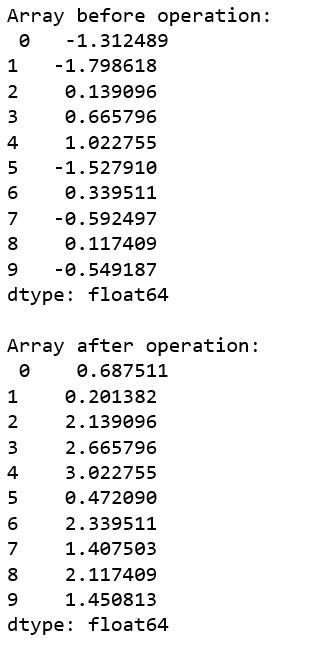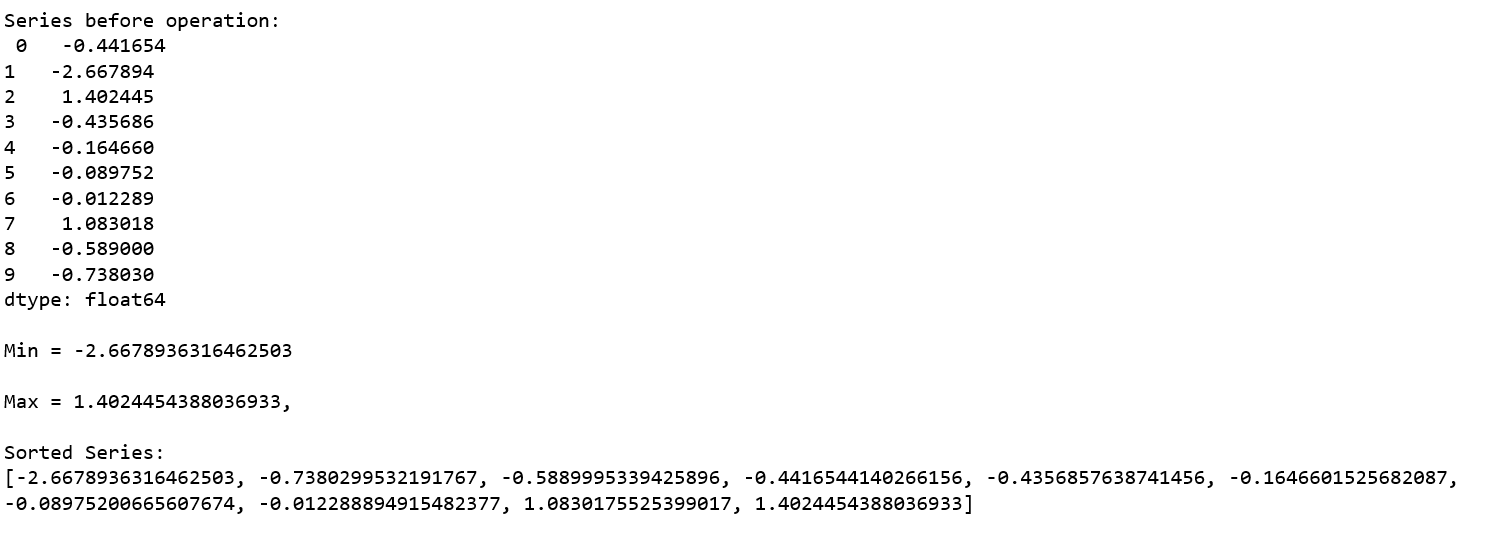# Python | Pandas Series.agg()

Python is a great language for doing data analysis, primarily because of the fantastic ecosystem of data-centric python packages. Pandas is one of those packages and makes importing and analyzing data much easier.

Pandas `Series.agg()` is used to pass a function or list of function to be applied on a series or even each element of series separately. In case of list of function, multiple results are returned by `agg()` method.

Syntax: Series.agg(func, axis=0)

Parameters:
func: Function, list of function or string of function name to be called on Series.
axis:0 or ‘index’ for row wise operation and 1 or ‘columns’ for column wise operation.

Return Type: The return type depends on return type of function passed as parameter.

Example #1:
In this example, a lambda function is passed which simply adds 2 to each value of series. Since the function will be applied to each value of series, the return type is also series. A random series of 10 elements is generated by passing array generated using Numpy random method.

 `# importing pandas module ` `import` `pandas as pd ` `  `  `# importing numpy module ` `import` `numpy as np ` `  `  `# creating random arr of 10 elements ` `arr``=``np.random.randn(``10``) ` `  `  `# creating series from array ` `series``=``pd.Series(arr) ` `  `  `# calling .agg() method ` `result``=``series.agg(``lambda` `num : num ``+` `2``)  ` `  `  `# display ` `print``(``'Array before operation: \n'``, series, ` `      ``'\n\nArray after operation: \n'``,result) `

Output:As shown in output, the function was applied to each value and 2 was added to each value of series.

Example #2: Passing List of functions

In this example, a list of some Python’s default function is passed and multiple results are returned by `agg()` method into multiple variables.

 `# importing pandas module ` `import` `pandas as pd ` `  `  `# importing numpy module ` `import` `numpy as np ` `  `  `# creating random arr of 10 elements ` `arr``=``np.random.randn(``10``) ` `  `  `# creating series from array ` `series``=``pd.Series(arr) ` `  `  `# creating list of function names ` `func_list``=``[``min``, ``max``, ``sorted``] ` `  `  `# calling .agg() method ` `# passing list of functions ` `result1, result2, result3``=` `series.agg(func_list)  ` `  `  `# display ` `print``(``'Series before operation: \n'``, series) ` `print``('\nMin ``=` `{}\n\nMax ``=` `{},\ ` `      ``\n\nSorted Series:\n{}'.``format``(result1,result2,result3)) `

Output:As shown in output, multiple results were returned. Min, Max and Sorted array were returned into different variables result1, result2, result3 respectively.

My Personal Notes arrow_drop_upCheck out this Author's contributed articles.

If you like GeeksforGeeks and would like to contribute, you can also write an article using contribute.geeksforgeeks.org or mail your article to contribute@geeksforgeeks.org. See your article appearing on the GeeksforGeeks main page and help other Geeks.

Please Improve this article if you find anything incorrect by clicking on the "Improve Article" button below.

Article Tags :

Be the First to upvote.

Please write to us at contribute@geeksforgeeks.org to report any issue with the above content.